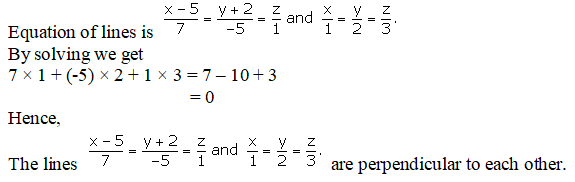# RD Sharma Solutions Class 12 Straight Line In Space Exercise 28.2

RD Sharma Solutions for Class 12 Maths Chapter 28 Straight Line in Space Exercise 28.2, is provided here for students to prepare for exams at ease. Practising the textbook questions will help you in analysing your level of preparation and knowledge of the concept. Students can download the RD Sharma Class 12 Solutions pdf for free from the links provided below.

## Download PDF of Rd Sharma Solution for Class 12 Maths Chapter 28 Exercise 2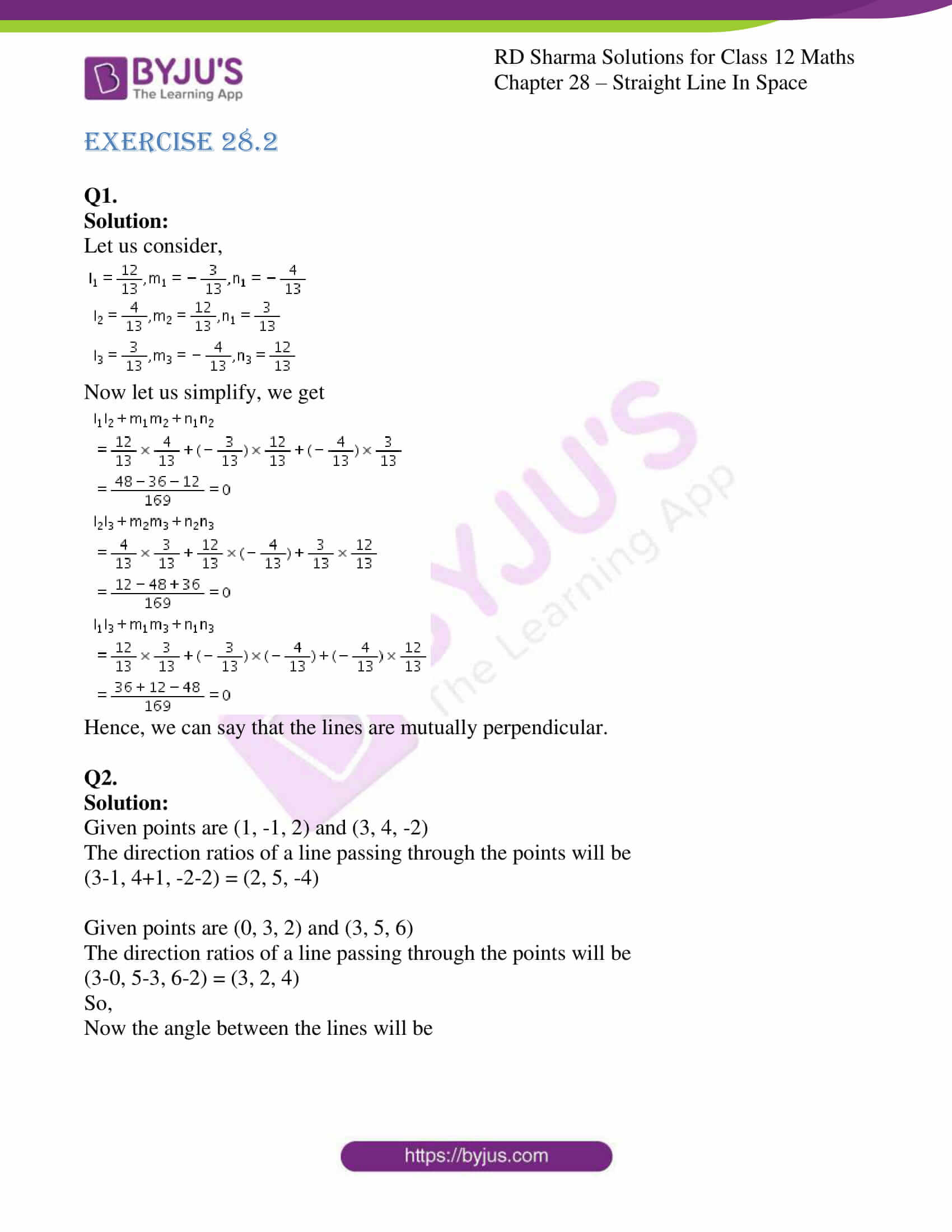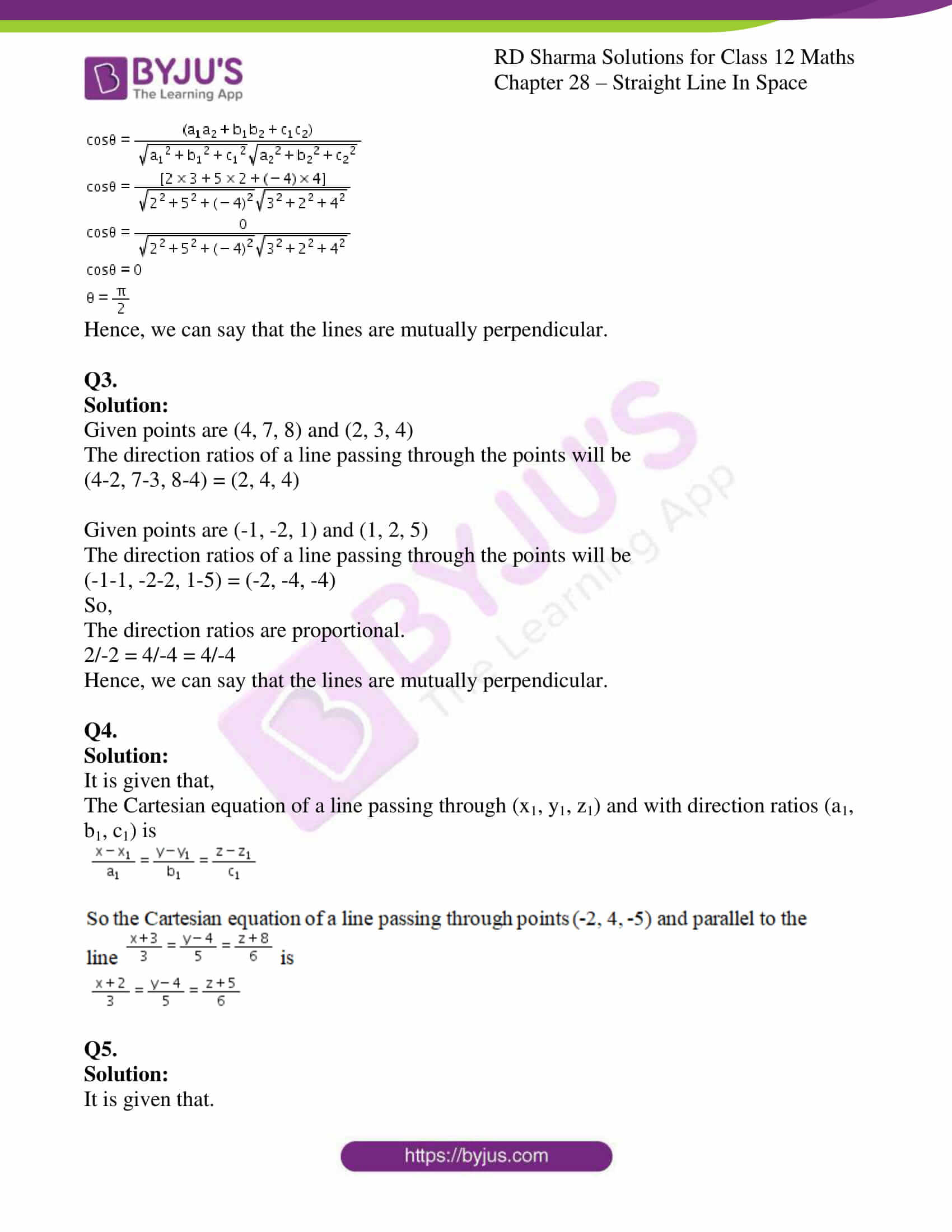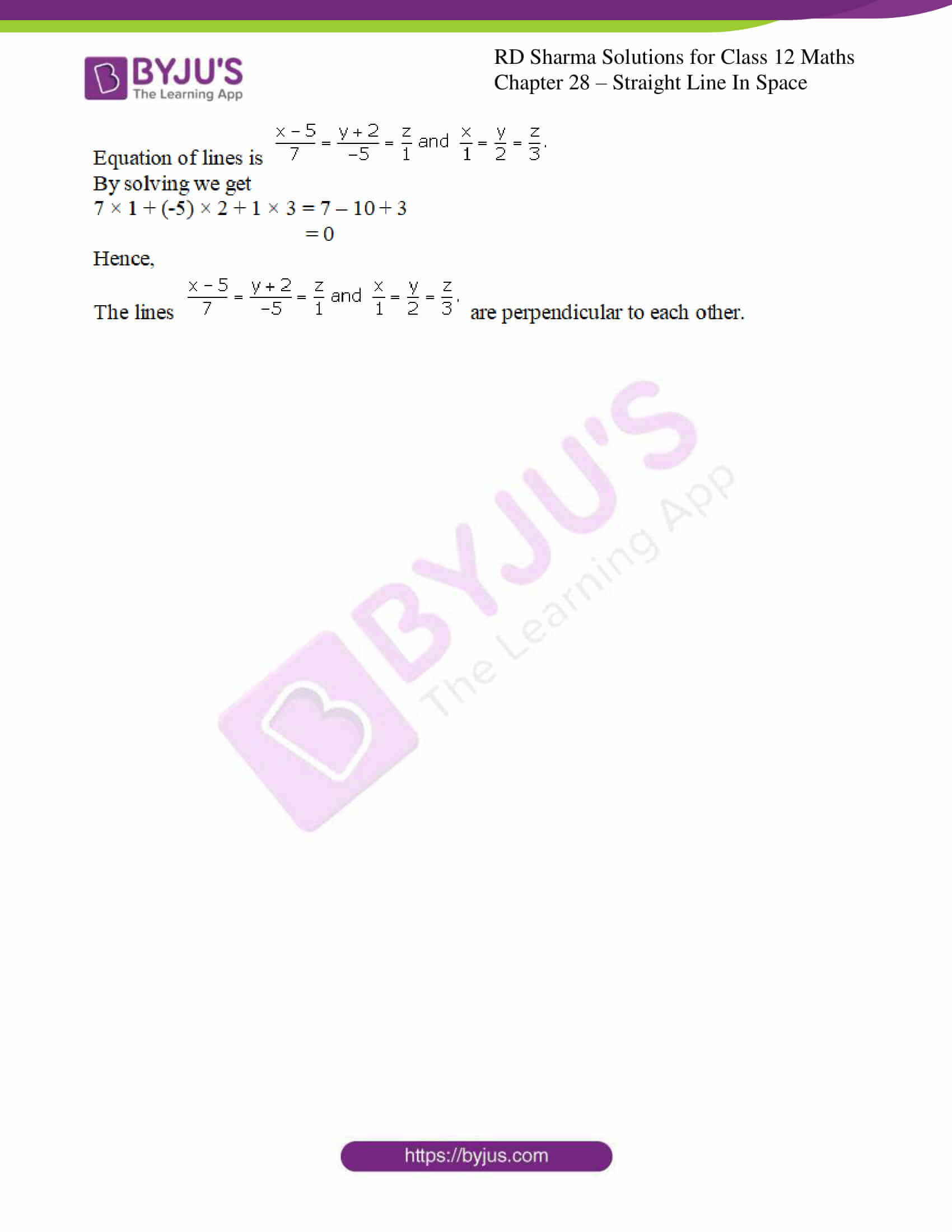### Access Answers for Rd Sharma Solution Class 12 Maths Chapter 28 Exercise 2

Q1.

Solution:

Let us consider,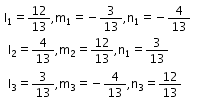Now let us simplify, we get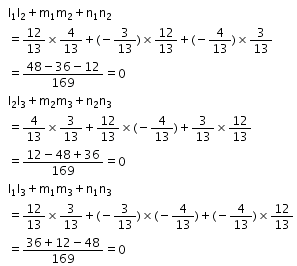Hence, we can say that the lines are mutually perpendicular.

Q2.

Solution:

Given points are (1, -1, 2) and (3, 4, -2)

The direction ratios of a line passing through the points will be

(3-1, 4+1, -2-2) = (2, 5, -4)

Given points are (0, 3, 2) and (3, 5, 6)

The direction ratios of a line passing through the points will be

(3-0, 5-3, 6-2) = (3, 2, 4)

So,

Now the angle between the lines will be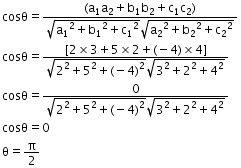Hence, we can say that the lines are mutually perpendicular.

Q3.

Solution:

Given points are (4, 7, 8) and (2, 3, 4)

The direction ratios of a line passing through the points will be

(4-2, 7-3, 8-4) = (2, 4, 4)

Given points are (-1, -2, 1) and (1, 2, 5)

The direction ratios of a line passing through the points will be

(-1-1, -2-2, 1-5) = (-2, -4, -4)

So,

The direction ratios are proportional.

2/-2 = 4/-4 = 4/-4

Hence, we can say that the lines are mutually perpendicular.

Q4.

Solution:

It is given that,

The Cartesian equation of a line passing through (x1, y1, z1) and with direction ratios (a1, b1, c1) is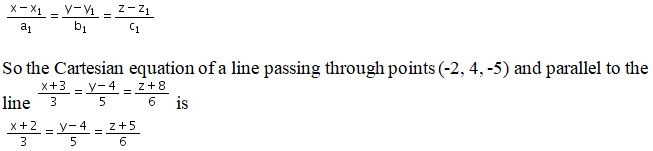Q5.

Solution:

It is given that.